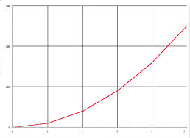# What is linearity?

Most potentiometers are linear output. That is, the theoretical relationship between mechanical travel and output voltage is linear. This measurement is called linearity.

Linearity is expressed as a percentage of total applied input voltage. As an example, a pot may have a linearity of 0.25%. This means that the actual output voltage, as the wiper is moved, would only deviate from the theoretical by only 0.25%.

Although there are many different kinds of linearity, the type referred to generally in the potentiometer industry is independent linearity.